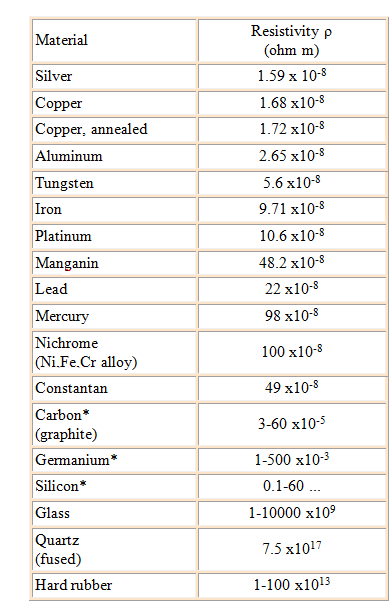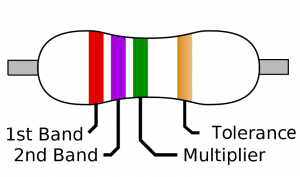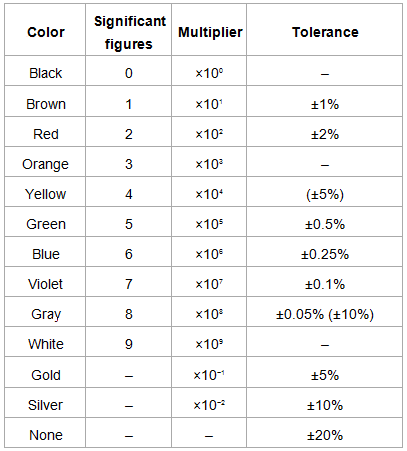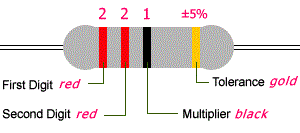# Resisitivity of Various Materials

Electrical Conductivity and Resistivity

Electrical conductivity is the ability of a material to conduct electricity. Electrical resistivity is the reciprocal of electrical conductivity. It is the measure of the ability of a material to oppose the flow of current.

• Metals are good conductors of electricity. Hence, they have low resistivity.
• The insulators like rubber, glass, graphite, plastics, etc. have very high resistivity when compared to the metallic conductors.
• The third type is the semiconductor which comes in between the conductors and insulators. Their resistivity decreases with the increase in temperature and is also affected by the presence of impurities in them.

The table below lists the electrical resistivity of several conductors, semiconductors, and insulators.### Resistor Color-Coding

Resistors are used in electrical circuits to control or reduce the flow of current in the circuit. Their resistance is indicated by using electronic color codes. Different color bands or rings are marked on these resistors for different values of resistance.Highlights of the resistor colour-coding are as follows:

• It normally contains four bands.
• The first band is indicative of the first significant figure of the resistance.
• The second band is the second significant figure. (At times there is a third band to have more precision and hence, they are 5 band resistors.)
• The third band is the decimal multiplier.
• The fourth band is indicative of the tolerance (in percentage) that the resistor can withstand about the indicated values.
• In the absence of the fourth band, a default tolerance of 20% is taken.

The table below lists various colours codes and their values in terms of significant figures, multiplier, and tolerance.Let us take an example to understand resistor colour coding.• The resistor has four colour bands.
• The first band is red. From the table, we know that red stands for the significant digit 2.
• The second is red too. This is indicative of the second significant digit 2.
• The third band is a multiplier and is black. For black, the multiplier is 100 or 1.
• The fourth band is gold which indicates a tolerance of ±5%.
• Hence, the resistor has a resistance of 22 x 1 Ω with ±5% tolerance.

To learn more about resistivity of various materials, download Byju’s The Learning App.

#### Practise This Question

Find the resistance of a hollow cylinder of inner radius a and outer radius b whose inner and outer cylindrical surfaces are connected by to a  p.d (ρ resistivity)